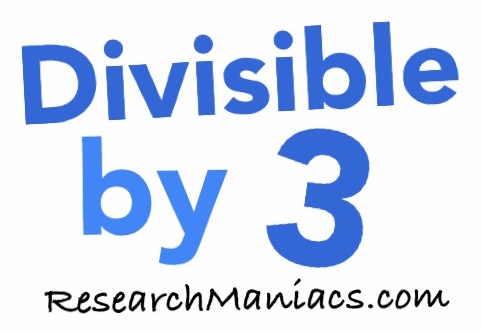Is 8 divisible by 3?Is 8 divisible by 3? In other words, if you divide 8 by 3, will you get a whole number with no remainder?

Of course, you could use a calculator to find out if 8 is divisible by 3, but what fun would that be?

To find out if 8 is divisible by 3, we will add up the numbers that make 8 as follows: 8 = 8

We know that if the sum of the numbers that make up 8 is divisible by 3, then 8 is divisible by 3.

Since the sum of the digits in 8 is not divisible by 3, 8 is also NOT divisible by 3. Thus, the answer to the question "Is 8 divisible by 3?" is as follows:

No

Note: If you divide 8 by 3, you get 2.67 which is not a whole number.

Divisible by 3?
Do you need to check another number? Enter a number below to see if it is divisible by 3.

Is divisible by 3?

Is 9 divisible by 3?
Can you do it on your own now? If not, go here for the next number on our list.